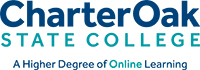Charter Oak State College Official Catalog

# Math

## MAT 100 - Elementary Algebra (1 credits)

This course will focus on the basics of algebraic notation and the foundation skills for higher-level mathematics courses. It is specifically designed for students who wish to refresh their algebra skills prior to or while simultaneously taking MAT 101, MAT 103, or MAT 105 at Charter Oak State College. Topics include: algebraic expressions, one-variable linear equations, slope and straight line formulas, graphs of linear equations and systems of linear equations, linear inequalities, and concepts of linear programming using systems of linear inequalities. This course cannot be used towards either the Math concentration or the Math general education requirements. Prerequisite(s): High school Algebra.

## MAT 101 - Contemporary Mathematics (3 credits)

This course will focus on the application of mathematics to today's world. Topics include street networks, producing and describing data, number and bar codes, weighted voting and apportionment, social choices and linear programming applications. Recommended Prerequisite(s): High school Algebra (2 years) or MAT 100 (may be taken concurrently).

## MAT 103 - College Algebra (3 credits)

This course will focus on a study of the fundamentals of college level algebra. Topics include: algebraic expressions, equations and inequalities, function theory, linear and quadratic functions, polynomials, rational and radical expressions, quadratic equations and inequalities, exponentials and logarithms, and the Conic Sections. Recommended Prerequisite(s): High school Algebra (2 years) or MAT 100 with a final grade of B or above.

## MAT 105 - Statistics (3 credits)

This course will focus on the fundamentals of modern statistics. Topics include: basic concepts of descriptive statistics, inferences about the mean, proportion, and variance of one population, the binomial distribution, confidence intervals, and hypothesis testing. Other topics in this course include an introduction to probability and bivariate data, including linear correlation and regression. This course is an Open Educational Resource Course. Recommended Prerequisite(s): High school Algebra (2 years) or MAT 100 (may be taken concurrently).

## MAT 115 - Logic (3 credits)

This course will focus on a study of formal and informal logic, deductive and inductive reasoning, hypothetical reasoning, valid argument forms, symbolic logic, sentential and predicate language, statement logic, tautologies, and the use of validity/invalidity in logic. Emphasis is placed on the proper uses of logic for problem-solving techniques.

OFFICIAL CATALOG 2021-2022 > Courses > Math Courses